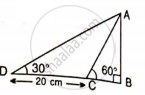# Find Ab and Bc, If: - Mathematics

Sum

Find AB and BC, if:#### Solution

Let  BC = x m
BD = BC + CD = (x + 20) cm

In ΔABD,
tan 30° = "AB"/"BD"

(1)/(sqrt(3)) = "AB"/(x + 20)

x + 20 = sqrt(3)"AB"      ...(1)

In ΔABC
tan 60° = "AB"/"BC"

sqrt(3) = "AB"/x
x = "AB"/sqrt(3)         ...(2)
From (1)

"AB"/sqrt(3) + 20 = sqrt(3)"AB"

AB + 20sqrt(3) = 3AB

2AB = 20sqrt(3)

2AB = (20sqrt(3))/(2)

AB = 10sqrt(3)

AB = 17.32 cm

From (2)

x = "AB"/sqrt(3)

x = (17.32)/sqrt(3)

x = 10 cm

Therefore BC = x = 10 cm
Therefore, AB = 17.32 cm, BC = 10 cm.

Concept: Solution of Right Triangles
Is there an error in this question or solution?

#### APPEARS IN

Selina Concise Mathematics Class 9 ICSE
Chapter 24 Solution of Right Triangles [Simple 2-D Problems Involving One Right-angled Triangle]
Exercise 24 | Q 17.2 | Page 304